Next: Global function fits Up: Nonlinear prediction Previous: Finding unstable periodic orbits

## Locally linear prediction

If there is a good reason to assume that the relation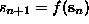is fulfilled by the experimental data in good approximation (say, within 5%) for some unknown f and that f is smooth, predictions can be improved by fitting local linear models. They can be considered as the local Taylor expansion of the unknown f, and are easily determined by minimizing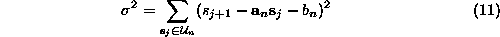with respect to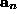and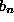, where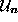is the-neighborhood of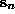, excludingitself, as before. Then, the prediction is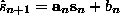. The minimization problem can be solved through a set of coupled linear equations, a standard linear algebra problem. This scheme is implemented in onestep. For moderate noise levels and time series lengths this can give a reasonable improvement over zeroth and predict. Moreover, as discussed in Sec., these linear maps are needed for the computation of the Lyapunov spectrum. Locally linear approximation was introduced in [45, 46]. We should note that the straight least squares solution of Eq.() is not always optimal and a number of strategies are available to regularize the problem if the matrix becomes nearly singular and to remove the bias due to the errors in the ``independent'' variables. These strategies have in common that any possible improvement is bought with considerable complication of the procedure, requiring subtle parameter adjustments. We refer the reader to Refs. [51, 52] for advanced material.

In Fig.we show iterated predictions of the Poincaré map data from the COlaser (Fig.) in a delay representation (using nstep in two dimensions). The resulting data do not only have the correct marginal distribution and power spectrum, but also form a perfect skeleton of the original noisy attractor. There are of course artefacts due to noise and the roughness of this approach, but there are good reasons to assume that the line-like substructure reflects fractality of the unperturbed system.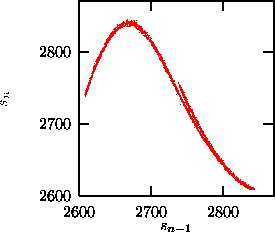Figure:   Time delay representation of 5000 iterations of the local linear predictor nstep in two dimensions, starting from the last delay vector of Fig..

Casdagli  suggested to use local linear models as a test for nonlinearity: He computed the average forecast error as a function of the neighborhood size on which the fit forandis performed. If the optimum occurs at large neighborhood sizes, the data are (in this embedding space) best described by a linear stochastic process, whereas an optimum at rather small sizes supports the idea of the existence of a nonlinear almost deterministic equation of motion. This protocol is implemented in the routine ll-ar, see Fig..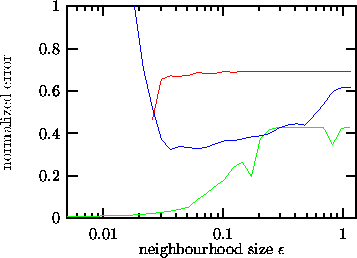Figure:   The Casdagli test for nonlinearity: The rms prediction error of local linear models as a function of the neighborhood size. Lower (green) curve: The COlaser data. These data are obviously highly deterministic in m=4 dimensions and with lag=6. Central (blue) curve: The breath rate data shown in Fig.with m=4 and=1. Determinism is weaker (presumably due to a much higher noise level), but still the nonlinear structure is dominant. Upper (red) curve: Numerically generated data of an AR(5) process, a linearly correlated random process (m=5,=1).Next: Global function fits Up: Nonlinear prediction Previous: Finding unstable periodic orbits

Thomas Schreiber
Wed Jan 6 15:38:27 CET 1999